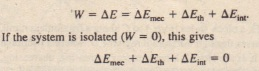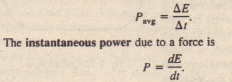# REVIEW & SUMMARY

REVIEW & SUMMARY

Conservative Forces A force is a conservative force if the net work it does on a particle moving around every closed path. from an initial point and then back to that point. is zero.

Potential Energy A potential energy is energy that is associated with the configuration of a system in which a conservative force acts. When the conservative force does work.

Gravitational Potential Energy The potential energy associated with a system consisting of Earth and a nearby particle is gravitational potential energy.

Elastic Potential Energy Elastic potential energy is the energy associated with the state of compression or extension of an elastic object.

Mechanical Energy The mechanical energy of a system. The change  is related to the magnitude  the frictional is the sum of its kinetic energy K and its potential energy.

Potential Energy Curves If we know the potential energy (unction U(x) for a system in which a one-dimensional force.

Work  on a System by an External Force Work W is energy transferred to or from a system by means of an external force acting on the system.

Conservation of Energy The total energy E of a system (the sum of its mechanical energy and its internal energies, including thermal energy) can change only by amounts of energy that are transferred to or from the system.The power due to a force is the rate at which that force transfers energy,